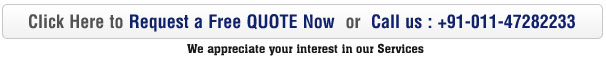# Multivariate Techniques: The Many Benefits And Some Drawbacks

Tips & Tutorial | Admin | Updated: 2012-12-27

Multivariate Techniques basically refer to research techniques that are used for determining the relationship between two or more variables. These techniques help derive the relationship between different variables, the correlation between the two. It also determines how the final result is based on these variables & how these variables affect the final result.

### Multivariate Techniques: Where All Is It Used

• Used in market research
• Used in R&D programs
• Used in quality control techniques
• Used in process optimization techniques

### Common Types Of Multivariate Techniques

• Multiple Regression
• Factor Analysis
• Path Analysis
• Multiple Analysis of Variance

### Benefits of Multivariate Techniques

• Multivariate Techniques help determine the relationship between different multiple variables in a quantifiable way. Through Multivariate Techniques one can determine how one variable affects the other & finally what effects the variables will have on the final outcome.
• By determining the relationship between multiple variables & the condition under which the links are made, one is in a better position to control the variables and change the outcome, wherever needed.
• Multivariate Techniques provide statistical account for the links between variable (whether dependent or independent), the conditions that control these formations, etc.

### Drawbacks Of Multivariate Techniques

• Multivariate Techniques are a highly complex science & analysis method. There are usually experts that understand these kinds of statistical analysis and highly complex mathematical data. The output of the data received through implementing Multivariate Techniques is complex & again require the services of an expert to make sense of it.
• Multivariate Techniques involve the use of complex statistical programs that are usually very expensive.
• To come to a proper conclusion & get accurate results, a large amount of a data is required for such kind of statistical data analysis.

But these negative points are not bad if compared to the many benefits of using Multivariate Techniques. Multivariate Techniques are an integral part of the research and development domain today & are widely implemented across many industries.